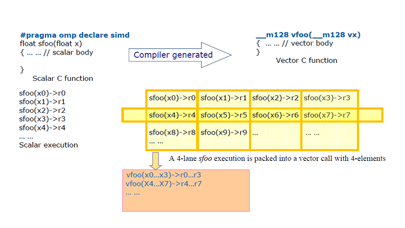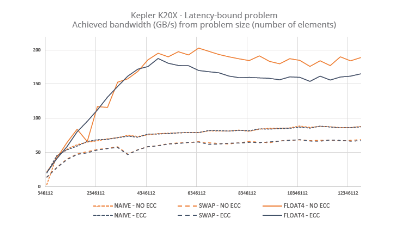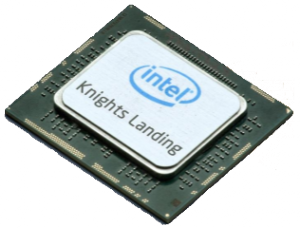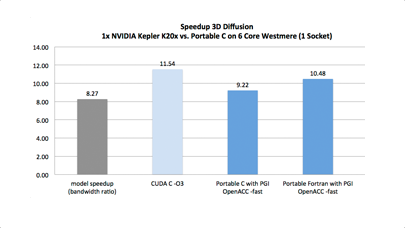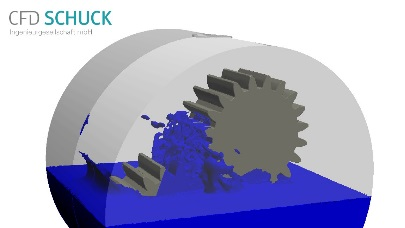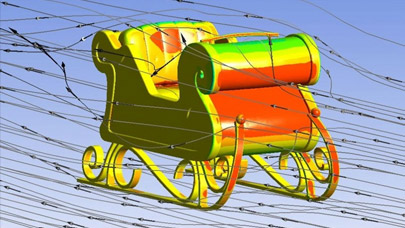Explicit Vector Programming with OpenMP 4.0 SIMD Extensions
By and   |  November 19, 2014

4 – EXPLICIT VECTOR PROGRAMMING EXAMPLES

This section provides several explicit vector programming examples in C/C++ and Fortran. In practice, compilers may not vectorize loops when they are complex or have potential dependencies, even though the programmer is certain the loop will execute correctly as a vectorized loop. Sophisticated compilers can do runtime tests and multi-versioning for simple cases, but the code size and the compile time increase. The `simd` construct can be used to address these issues. Application programmers can use it to assure the compiler that the loop can be vectorized.

The example in listing 4.1 (written in both OpenMP C++ and Fortran) shows that `*ioff` is unknown at compile time, so the compiler has to assume `*ioff` could be either a negative or a positive integer value for each invocation of function `star`. Also the compiler does not know if `a`, `b` and `c` are aliased. For example, if `a` and `c` are aliased and `*ioff = -2`, then this loop has a loop-carried lexically backward dependency, so it is not vectorizable. However, if a programmer can guarantee `*ioff` is a positive integer, the loop can be vectorized even if `a` and `c` are aliased. The programmer can use a `simd` construct to guarantee this property.

```void star( double *a, double *b, double *c, int n, int *ioff ) {
<strong>#pragma omp simd</strong>
for (int i = 0; i < n; i++) {
a[i] *= b[i] * c[i+ *ioff];
}
}```
```subroutine star(a, b, c, n, ioff_ptr)
implicit none
double precision :: a(:),b(:),c(:)
integer          :: n, i
integer, pointer :: ioff_ptr
<strong>!\$omp simd</strong>
do i = 1,n
a(i) = a(i) * b(i) * c(i+ioff_ptr)
end do
end subroutine```

listing 4.1 – A loop example written in C++ and FORTRAN.

The second example (written in both OpenMP C/C++ and Fortran) in listing 4.2 shows that the `simd` construct can be used for a recursive function. The function `fib` is called unconditionally in main and also conditionally and recursively in itself. By default, the compiler would create a masked vector version and a non-masked vector version while retaining the original scalar version.

```#include <stdio.h>
#include <stdlib.h>
#define N 45
int a[N], b[N], c[N];
<strong>#pragma omp declare simd inbranch</strong>
int fib(int n) {
if (n <= 2)
return n;
else {
return fib(n-1) + fib(n-2);
}
}
```
```int main(void) {
for (int i=0; i < N; i++) b[i] = i;
<strong>#pragma omp simd</strong>
for (int i=0; i < N; i++) {
a[i] = fib(b[i]);
}
printf("Done a[%d] = %dn", N-1, a[N-1]);
return 0;
}
</code><code>
program Fibonacci
implicit none
integer,parameter :: N=45
integer           :: a(0:N-1), b(0:N-1)
integer, external :: fib
do i = 0,N-1
b(i) = i
end do
<strong>!\$omp simd</strong>
do i=0,N-1
a(i) = fib(b(i))
end do
write(*,*) "Done a(", N-1, ") = ", a(N-1)  ! 44  1134903168
end program
```
```recursive function fib(n) result(r)
<strong>!\$omp declare simd(fib) inbranch</strong>
implicit none
integer  :: n, r
if (n <= 2) then
r = n
else
r = fib(n-1) + fib(n-2)
endif
end function fib```

listing 4.2 - A loop example using declare simd and simd construct.

Although the call to `fib` in `main` is not under the condition, the programmer can manually inline the top level call in the loop to `fib`, which would allow the use of the `inbranch` clause. Most modern compilers would perform this inlining automatically so `fib` is always called under the condition, as the example assumes. Either choice would instruct the compiler to generate only the masked vector version, which would reduce compile time and code size.

<12345678>

More around this topic...

In the same sectionBy the same authorThank you for reading HPC Today.

Express poll

Do you use multi-screen
visualization technologies?

Industry news

Trending this week...